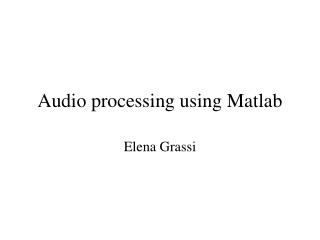DownloadDownload PresentationAudio processing using Matlab

# Audio processing using Matlab

Télécharger la présentation## Audio processing using Matlab

- - - - - - - - - - - - - - - - - - - - - - - - - - - E N D - - - - - - - - - - - - - - - - - - - - - - - - - - -
##### Presentation Transcript

1. Audio processing using Matlab Elena Grassi

2. Sampling • Read values from a continuous signal • Equally spaced time interval (sampling frequency)

4. Spectrogram • Short time Fourier transform • Tradeoff frequency/time resolution. Note: dB= 20*log10 () specgram(y, 256, fs) title('Spectrogram [dB]')

5. D/A (digital in/analog out) AO = analogoutput('winsound'); addchannel(AO,1); set(AO,'SampleRate',22050) set(AO,'TriggerType','Manual') putdata(AO,x) start(AO) trigger(AO) waittilstop(AO,5) delete(AO), clear AO

6. Aliasing • When sampling is too slow for a signal’s BW, high frequency content cannot be observed and it leaks into lower frequencies, thus distorting the signal. • Minimum sampling required to capture the signal accurately: Nyquist frequency= 2*BW • If not possible, apply antialiasing filter.

7. Filters Modify frequency content of signals. Classification according to their pass/stop bands: • Lowpass (smoothing filter) • Highpass • Bandpass • Stopband Specify corner frequency(ies), normalized wrt ½ sampling frequency. Example: 2000/(fs/2) for 2000 Hz.

8. Example

9. Filter Types Classification according to their roll-off, flatness, phase: • Bessel: linear phase, preserves wave shape. • Butterworth: flat and monotonic, sacrifice roll-off steepness. • Chebyshev I: equiripple in passband and monotonic in stopband. • Chebyshev II: monotonic in passband and equiripple in stopband, roll off slower than type I.

10. Example [b,a]= butter(6,2000*2/fsi,'low'); sampling freq corner freq order b= numerator polynomial in z a= denominator polynomial in z

11. Filter frequency response h= impz(b,a,N); H=(abs(fft(h))); fscale= fsi/N*(1:N/2); plot(fscale,H(1:N/2),'r') xlabel('f [Hz]') title('Filter frequency response')

12. Filter order • Related to complexity (hardware or numerical) and how many samples of data are used. • Higher order <-> Steepness • Trade off with complexity/numerical stability